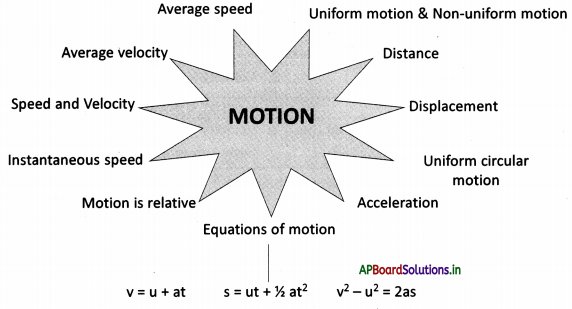# AP Board 9th Class Physical Science Notes Chapter 1 Motion

Students can go through AP State Board 9th Class Physical Science Notes Chapter 1 Motion to understand and remember the concept easily.

## AP State Board Syllabus 9th Class Physical Science Notes Chapter 1 Motion

→ Right and left, day and night, up and down, larger and shorter are relative terms with respect to the position, and point of view of the observer

→ Motion is relative to the observer.

→ A body is said to be In motion when Its position is changing continuously with time relative to the observer.

→ Distance is the length of the path traversed by an object in a given time Interval.

→ Displacement is the shortest distance covered by the object in a specified direction. Its unit in the SI system is ‘meter’.

→ Distance is a scalar quantity, whereas displacement is a vector quantity.

→ Average speed is the distance covered in unit time.

→ Average velocity is the displacement of an object per unit of time.

→ The speed at any instant is called instantaneous speed.

→ Distance -vs- time graph is more useful to study the motion of an object along a straight line, (time on X-axis, distance on Y-axis)

→ The slope of the curve in the D -t graph, at any point gives the speed of the object at that instant.→ If a body moves in a curved path, the tangent drawn at a point on the curve gives the direction of velocity at that instant.

→ The motion of the body is said to be uniform when its velocity is constant.

→ D -t graph for a body that is in uniform motion in a straight line.

→ The motion of the body is said to be non-uniform when its velocity changes from time to time.

→ Acceleration is the rate of change of velocity a = $$\frac{\Delta v}{\Delta t}$$

→ The SI unit of acceleration is m/sec2.

→ If the rate of change of velocity is negative, then it is called deceleration (Retardation) denoted by ‘-a’.

→ Equations of uniform accelerated motion are
v = u + at …………………(1)
s = ut + $$\frac{1}{2}$$ at2 ……………..(2)
v2 – u2 = 2as ……………..(3)

→ Relative: That exists or that has a particular quality only when compared with something else. Ex: The position of the sun is relative to the earth.→ Distance: The length between any two points in a given direction.

→ Displacement: Displacement is the shortest distance covered by the object in a specified direction.

→ Average speed: The distance covered in unit time is called average speed.
Average speed = $$\frac{\text { Total distance }}{\text { Time taken }}$$

→ Average velocity: The displacement of an object per unit time is called average velocity.
Average velocity = $$\frac{\text { Displacement }}{\text { Time taken }}$$

→ Instantaneous speed: Speed at an instant is instantaneous speed which gives the idea of how fast the position of the body changes.

→ Speed: Speed = $$\frac{\text { Distance }}{\text { Time }}$$

→ Velocity: Velocity = $$\frac{\text { Displacement }}{\text { Time }}$$

→ Uniform motion: The motion of the body is said to be uniform when its velocity is constant.

→ Acceleration : Acceleration is the rate of change of velocity.
Acceleration a = $$\frac{\Delta \mathrm{v}}{\Delta \mathrm{t}}$$. The SI unit of acceleration is m/sec2.

→ Uniform acceleration: The motion of an object along a straight line with constant acceleration.
(OR)
Equal chances of velocity occur In equal Intervals of time.→ Rectilinear motion: The motion of a body along a straight line is called rectilinear motion.

→ Deceleration: It is the decreasing velocity of the object.→ Aristotle:

• Aristotle was born in 384 BC and died in 322 BC.
• He was a Greek ancient philosopher.
• Aristotle saw ultimate reality in physical objects, knowable through experience.